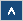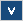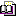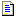Verilog Online HelpTable of ContentsBit-selectBlock StatementsBuilt-in PrimitivesCase StatementContinuous AssignmentsConversion FunctionsCommentsCompiler DirectivesConcatenationsConditional OperatorDelaysDisable StatementDisplay TasksEdge Sensitive PathExpression Bit LengthFile I/O FunctionsFunctionsIdentifiersIf StatementInteger ConstantsIntra-assignment Timing ControlsKeywordsLoop StatementsMemoriesmin:typ:max DelaysModule DeclarationModule InstantiationModule Path DeclarationModule Path PolarityNet Data TypesOperatorsParametersPart-selectPLA Modeling TasksProbabilistic Distribution FunctionsProcedural AssignmentsProcedural Continuous AssignmentsProcedural Timing ControlRange SpecificationReal ConstantsRegister Data TypesSimulation Control TasksSimulation Time FunctionsSpecify BlockState Dependent PathStochastic Analysis TasksStrengthsStringsStructured ProceduresTasksTimescale System TasksTiming Check TasksUDP DeclarationUDP InstantiationUDP State TableValue Change Dump (VCD) FileVectors

## Formal Definition

System tasks display specific information from the simulator.

## Simplified Syntax

\$display | \$displayb | \$displayh | \$displayo (arguments) ;

\$write | \$writeb | \$writeh | \$writeo (arguments) ;

\$strobe | \$strobeb | \$strobeh | \$strobeo (arguments) ;

\$monitor | \$monitorb | \$monitorh | \$monitoro (arguments) ;

\$monitoron ;

\$monitoroff ;

## Description

Generally, display system tasks are grouped into three categories. The first one includes the display and write tasks such as the \$display and the \$write tasks. The second category is strobe monitoring, which consists of the \$strobe group of tasks and the continuous monitoring tasks such as the \$monitor task.

The first group of displaying tasks is very similar to print the function in the ANSI C language (the syntax is almost the same). The \$write and the \$display tasks work in the same way and the only difference is that the \$display task adds a new line character at the end of the output, while the \$write task does not.

When one of these tasks is invoked, it simply prints its arguments. The order of printed arguments is the same as the order that the x appear in the argument list. If no argument is specified, it can be declared a null argument (two adjacent commas) and when the display task is invoked, it simply prints a single space character (Example 1). An argument can be an expression that returns a value and a quoted string (see Example 2).

When an argument is given as a quoted string, there are several rules concerning format, the string argument is described in more detail in the String chapter. However there are some differences and extensions to format strings in display system tasks.

The display tasks have a special character (%) to indicate that the information about signal value is needed. When using a string, the compiler recognizes the % character and knows that the next character is a format specification. For a full description of all format specifications see the following table. If the format specification sign is used it should always be followed by a corresponding argument (exception is the %m argument).

If we need to display the % character we should use double %%.

 %d or %D Decimal format %b or %B Binary format %h or %H Hexadecimal format %o or %O Octal format %c or %C ASCII character format %v or %V Net signal strength %m or %M Hierarchical name %s or %S As a string %t or %T Current time format

Table 1 The format specification for display system tasks.

When the \$display or \$write system task is executed it displays the string, and all format specifications are replaced with corresponding expression values (see Example 3 and 4).

These system tasks can be invoked with "o", "h" and "b" extensions. For example \$writeb, \$writeo, and \$displayh. When invoked, they inform the simulator that there are some arguments without corresponding format specifications and the default display format should be changed. By default, \$display and \$write system tasks use the decimal format to change display formats (see Example 5).

For information on how the %t format specification works see the Timescale chapter. This format specification is similar to \$timeformat system task.

The size of the displayed data is very important. Generally it depends on the format specification. If you are using a hexadecimal format, the data will be displayed as four characters, each of them representing four bits of value (a single hexadecimal value can be represented as four bits). Similarly, octal values will be displayed as group of characters representing three bits. See Examples 4 and 5 for further information. The result of an expression is automatically sized, however you can change default settings by adding 0 (zero) after the % character. Example 6 shows how it is applied and displays the results. In the decimal format, values with leading zeros will be replaced by a space character. The number of spaces added corresponds to the number of truncated zeros.

Another very useful display tasks feature is rules applying to the result of an expression that has an unknown or high impedance value.

If you are using decimal format (%d) the following rules apply:

• Single lowercase "x" character will be displayed when all bits are of an unknown value

• Single uppercase "X" character will be displayed when some bits are of an unknown value

• Single lowercase "z" character will be displayed when all bits are of a high impedance value

• Single uppercase "Z" character will be displayed when some bits are of a high impedance

If you are using hexadecimal (%h) and octal (%o) formats the following rules apply:

• Single lowercase "x" will be displayed when all bits in group are of an unknown value

• Single uppercase "X" will be displayed when some bits in group are of an unknown value

• Single lowercase "z" will be displayed when all bits in group are of a high impedance value

• Single uppercase "Z" will be displayed when some bits in group are of a high impedance

 NOTE: In the octal format, a group represents a group of three bits that can be represented as one digit within the range is 0 to 7. In the hexadecimal format, a group of four bits can be represented as one character within the range 0 to 9 and characters in range a to f. (See Examples 7 and 8)

The strength information may be needed when dealing with nets. For this purpose there is a special %v format specification in Verilog HDL to retrieve such information. It reports strength of a net but only in a scalar type. Three characters represent the strength, which is displayed in the console. The first two characters inform us about strength and the third character is the actual value of the net. In Verilog there are only four values: 0, 1, x, z, but the value returned by %v can also be L or H. See the table below for a full description of the strength identifier:

The first two characters:

 Mnemonic Strength name Strength level Su Supply drive 7 St Strong drive 6 Pu Pull drive 5 La Large capacitor 4 We Weak drive 3 Me Medium capacitor 2 Sm Small capacitor 1 Hi High impedance 0

The third character:

 0 Logic 0 value 1 Logic 1 value X An unknown value Z A high impedance value L Logic 0 or high impedance value H Logic 1 or high impedance value

For further rules on displaying strengths, refer to the chapter regarding Strength.

The second category of display tasks is the strobe monitoring tasks. The \$strobe task also has extensions such as o, b and h. They work in the same way as the \$display task, however when the \$display or \$write task is encountered, it is executed immediately. The \$strobe task is suspended until all events that occur in a particular time are processed. This means that this task returns values that are used in the next time unit. See example 10.

The last category of display tasks consists of continuous monitoring tasks. This category includes \$monitor, \$monitoron and \$monitoroff. The difference between this group of tasks and other tasks is that these tasks provide a means of displaying a value when an event occurs. The \$strobe returns value when all events in the time unit occurs, while \$monitor is sensitive to an event on a variable in its argument list.

## Examples

Example 1

\$display(, ,) ;
\$write(, ,) ;

These tasks produce single space characters; however \$display adds a new line character at the end of a line.

Example 2

reg a,b ;
\$display("Simple string");
\$write(a*b) ;

\$display produces a Simple string text and \$write evaluates a value of an expression and prints the result in the console window.

Example 3

// reg a, b ;
// a = 0 ;
// b = 1 ;
\$display("The value of a is: %b", a) ;
\$write ("The value of b is: %b", b) ;

The console window displays the following:

The value of a is: 0

The value of b is: 1.

Notice that the \$write task prints the results below the \$display tasks. (\$display adds a new line character). If you change the order of these tasks to make the \$write task first, then the console window will display the following:

The value of b is: 1 The value of a is: 0

This is because the \$write task does not add a new line character at the end of the line.

Example 4

reg [8:0] a ; // a = 492 ;
reg [7:0] b ; // b = 205 ;
\$display("The decimal value of a is: %d", a) ;
\$display("The octal value of a is: %o", a) ;
\$display("The binary value of a is: %b", a) ;
\$display("The hexadecimal value of a is: %h", a) ;
\$display("The decimal value of b is: %d", b) ;
\$display("The hexadecimal value of b is: %h", b) ;
\$display("The binary value of b is: %b", b) ;
\$display("The octal value of b is: %o", b) ;

The results are:

The decimal value of a is: 492

The octal value of a is: 754

The binary value of a is: 111101100

The hexadecimal value of a is: 1ec

The decimal value of b is: 205

The hexadecimal value of b is: cd

The binary value of b is: 11001101

The octal value of b is: 315

In the hexadecimal format, a single character represents a group of four bits and in the octal format , a single character represents a group of three bits.

Example 5

reg [8:0] a ; // a = 492 ;
\$display(a, ";") ;
\$displayb(a, ";") ;
\$displayo(a, ";") ;
\$displayh(a, ";") ;

The results are:

492;

111101100;

754;

1ec;

Example 6

reg [31:0] a ; // a = 40 ;
\$display("Decimal value a is: '%d'", a) ;
\$display("Decimal value a is: '%0d'", a) ;
\$display("Octal value a is: '%o'", a) ;
\$display("Octal value a is: '%0o'", a) ;

The results are:

Value a is: ' 40'

Value a is: '40'

Octal value a is: '00000000050'

Octal value a is: '50'

Notice that in the first case, the value is preceded by eight space characters. The register can store a maximum value of 4294967295. To print this number, 10 characters are needed. If only 2 characters are displayed (40), the remaining eight characters are replaced by space characters. By using the %0d format specification, you cause the value to be printed without any space characters and automatically resized. Space characters only in the case of decimal format replace the leading zeros. In other cases, zeros are always displayed.

Example 7

reg [4:0] a ;
\$display("Value a: %d", a) ; // a = 5'bxxxxx ;
\$display("Value a: %d", a) ; // a = 5'b1x1x0 ;
\$display("Value a: %d", a) ; // a = 5'bzzzzz ;
\$display("Value a: %d", a) ; // a = 5'b10zz1 ;
\$display("Value a: %d", a) ; // a = 5'b1x0zz ;

This example shows how the rules apply to %d format specification.

Results are:

Value a: x

Value a: X

Value a: z

Value a: Z

Value a: X

Notice that in the last case, the unknown bit and high impedance bits result in a single uppercase X. This is because an unknown value has higher priority than a high impedance value.

Example 8

reg [4:0] a ;
\$display("Value a: %o", a) ; // a = 5'bxxxxx ;
\$display("Value a: %o", a) ; // a = 5'b1x1x0 ;
\$display("Value a: %o", a) ; // a = 5'bzzzzz ;
\$display("Value a: %o", a) ; // a = 5'b10zz1 ;
\$display("Value a: %o", a) ; // a = 5'b1x0zz ;

For the %o format specification the results are:

Value a: xx

Value a: XX

Value a: zz

Value a: 2Z

Value a: XZ

See the expression bit length chapter for more details on zz and xx .

Example 9

reg [4:0] a ;
\$display("Value a: %h", a) ; // a = 5'bxxxxx ;
\$display("Value a: %h", a) ; // a = 5'b1x1x0 ;
\$display("Value a: %h", a) ; // a = 5'bzzzzz ;
\$display("Value a: %h", a) ; // a = 5'b10zz1 ;
\$display("Value a: %h", a) ; // a = 5'b1x0zz ;

The results are:

Value a: xx

Value a: 1X

Value a: zz

Value a: 1Z

Value a: 1X

Example 10

reg [4:0] a ;
\$strobe("Value a: %h", a) ;

## Important Notes

• To display the % character use double %%.